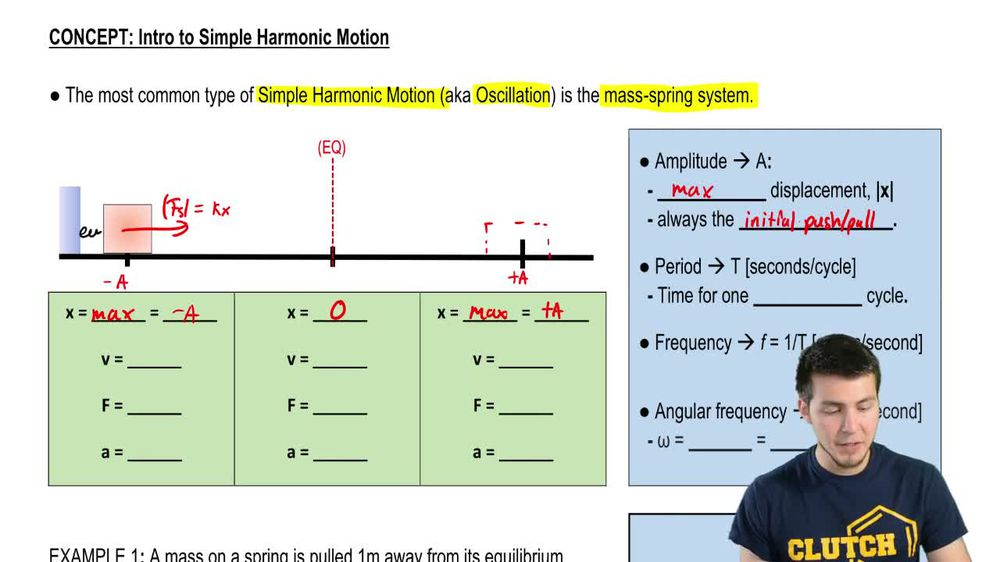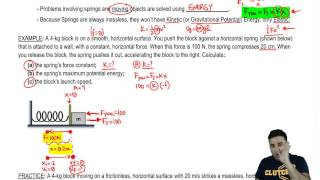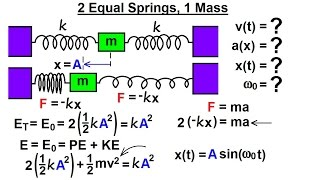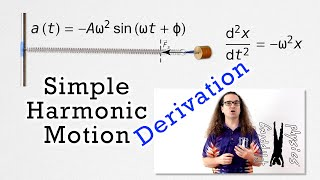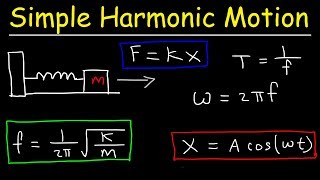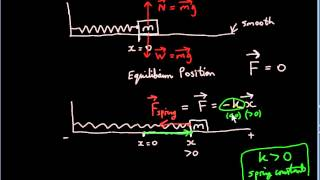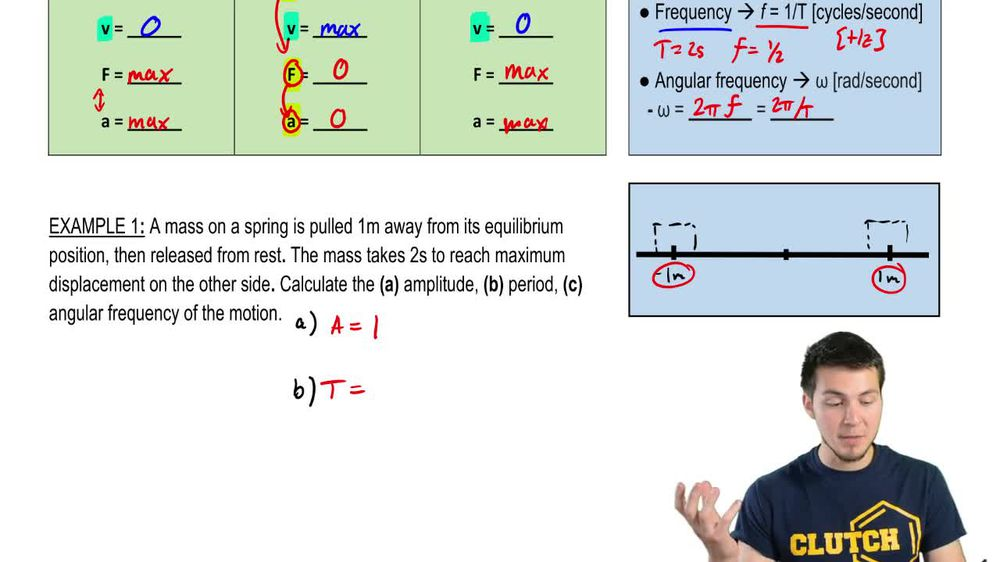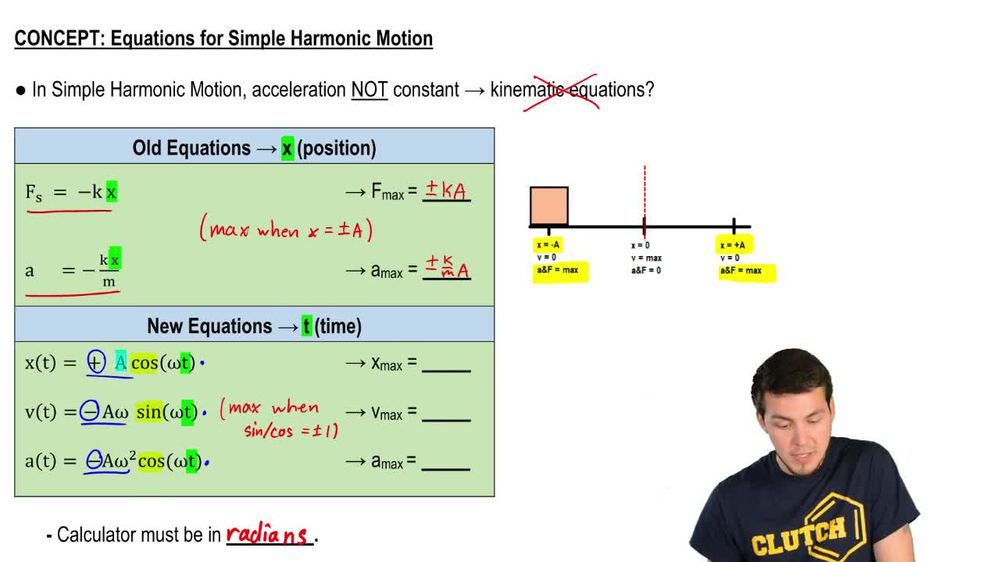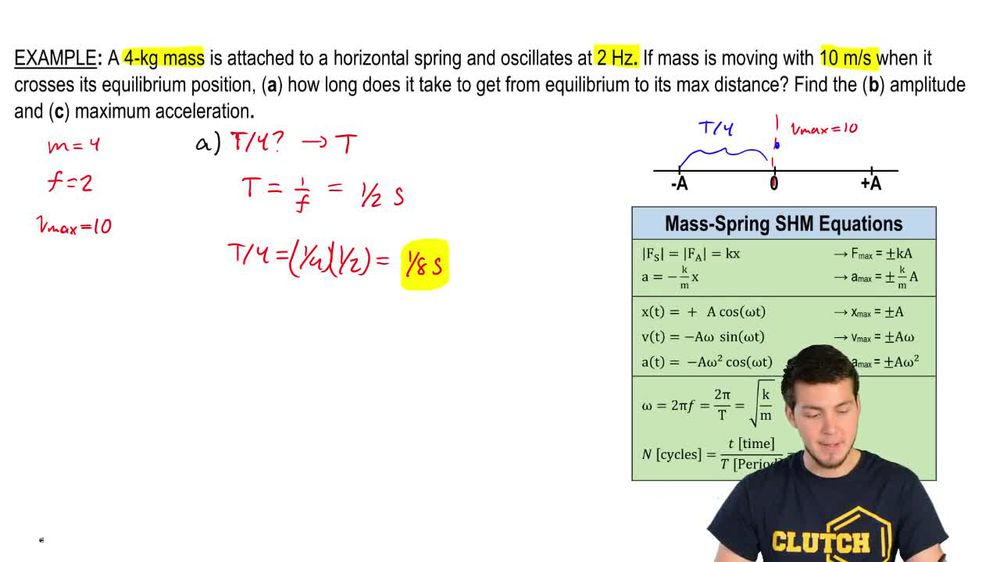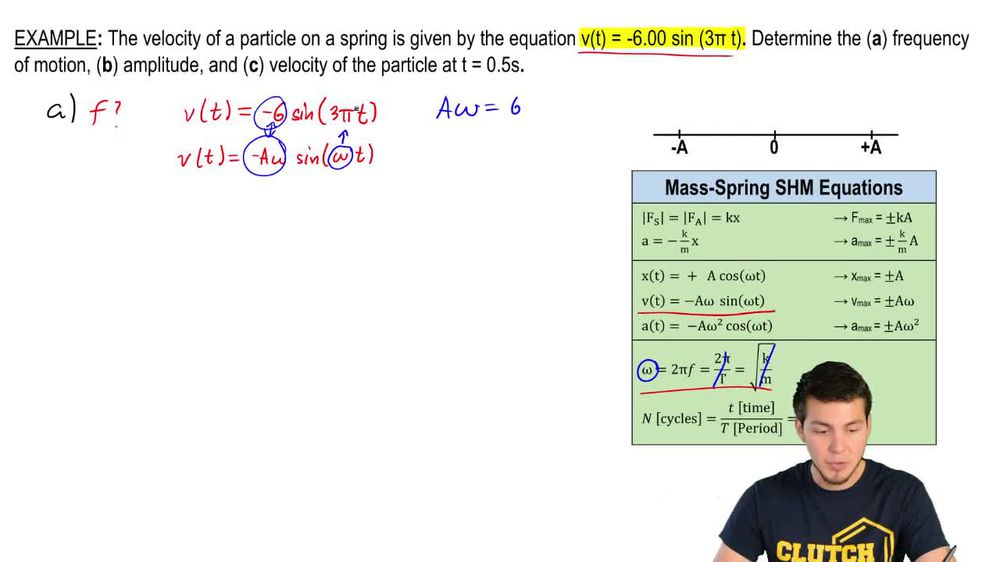Start typing, then use the up and down arrows to select an option from the list.
1. 17. Periodic Motion2. Intro to Simple Harmonic Motion (Horizontal Springs)# Example

by Patrick Ford
94 views
2
2
2
Hey, guys, let's take a look at this example. So we're told that the velocity of a particle is given by this equation, and that's the only thing that were given were supposed to find out what the frequency of motion is. So what they're asking us is to figure out what f is. So let's take a look at my equation list. The Onley equation that involves frequency is the big Omega equation right here. The problem is, I don't have any of those other variables. I don't have k over em. I don't have the period. And I don't know what the Omega is either. The only thing I am given is just the velocity equation. So let's take a look at the actual velocity equation with all the variables involved. So I've got the velocity is equal to negative six times the sign of three pi times T. But the form of the equation is I have negative a omega, Then I've got sign of omega times t. So if you just look at this, what is this telling us? Well, we've got this negative six out here and I've got a negative a omega, so that means that these things are equal to each other. So that means I have that a omega is equal to six. And if you look inside the parentheses, I've got this omega over here that's equal to this three pie. So those two things are the same. So that means that Omega, what they're telling me is equal to three pie. So now let's take a look at my frequency equation. I was I don't have the period and I don't have the square of Cavor em. But now I do have the Omega. So let's relate that equation eso I've got Omega is equal to two pi times the frequency. But omega, I just figured out was three pie. So I'm gonna set these two things equal to each other. So two pi times the frequency equals three pie. And now the frequency is just three pi over two pi. What will happen is the pies will cancel. And so I get frequency is equal to three halves. And that's the answer. That is the frequency of oscillation. So you just have to look at the equation and compare those variables to, like the actual form of the equation. to figure out what those things are. So let's look at part B. Part B is now asking us for the amplitude so great. So how do we find out the amplitude? We've got a bunch of equations that involved the amplitude Eso Let's take a look. I'm not told the X Max V Max or a Max. I'm never told any of those things and I don't know what the IMAX or the restoring forces, so I can't use that. So I'm gonna have to again look at this equation right here and from this equation, I figured out that a Omega was equal to six. So let's write that out. If a Omega is equal to six, then a is just going to be six divided by omega. But I figured out what Omega was. Well, remember that Omega was just equal to three times pi right over here. So that means that the amplitude is just too divided by pi once you go ahead and sold that. So I've got that's the amplitude to over pie. Now, for this last one, I'm supposed to find out what the velocity is at a specific time. So this is a question where they're giving me tea and I've asked for V, so I'm just gonna basically plugged in into my formula. So the velocity when t is equal to 0.5 seconds is gonna be negative six times the sign of three pi times 0.5. Make sure that you're in radiance mode and should get a velocity that's equal to 6 m per second, and that's it. Let me know if you guys have any questions, and if not, let's keep going.